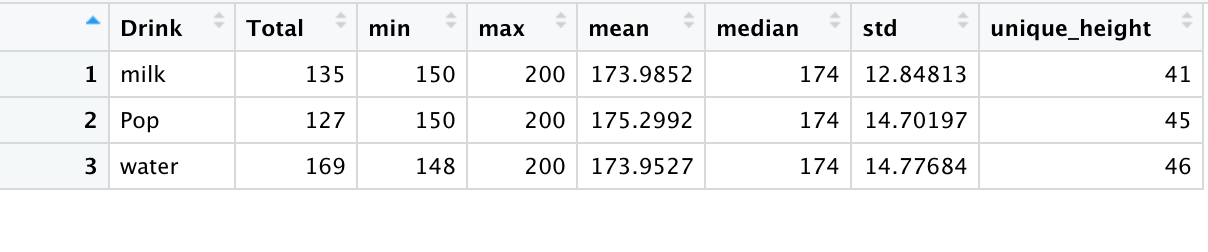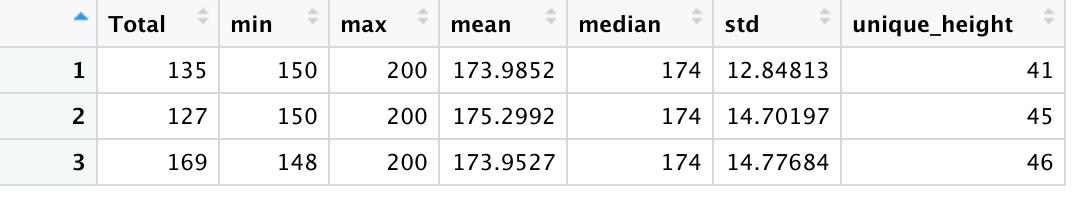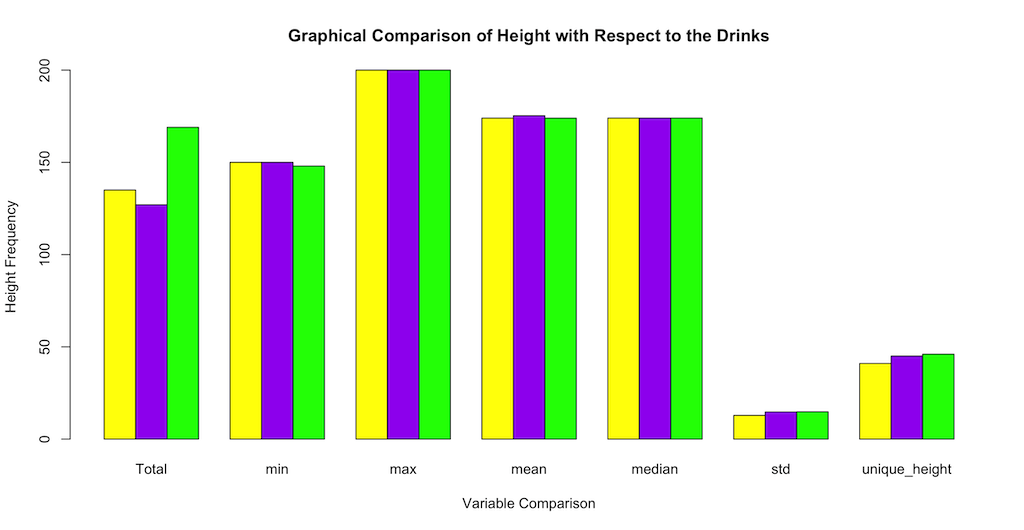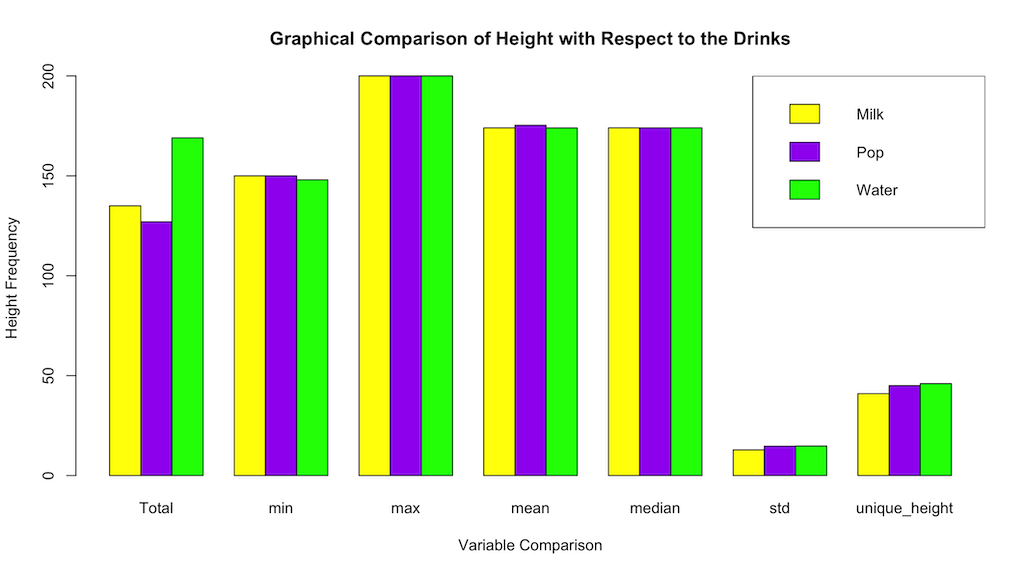is below this banner.

Can't find a solution anywhere?

NEED A FAST ANSWER TO ANY QUESTION OR ASSIGNMENT?

You will get a detailed answer to your question or assignment in the shortest time possible.

## Solution Using R Programming

This question was solved using R Studio.

We need to install two packages on R-Studio: xlsx (which allows the use of excel files on R programming) and dplyr (for easy data summary capability).

First, we will check if the two packages are already installed.

The code below checks if "xlsx" is installed. If installed, the output will be TRUE, otherwise, it'll be FALSE.

The code below checks if "dplyr" is installed. If installed, the output will be TRUE, otherwise, it'll be FALSE.

If your output is TRUE for both, you can skip this part. If it's false, run the codes below to install the two packages.

To install "xlsx":

To install "dplyr":

After the installation, to use both packages, you need to load them into the current runtime:

Use the code below to load the "xlsx" package:

Use the code below to load the "dplyr" package:

Having handled that, we can proceed to answer the question.

In the first part, we will select a categorical variable from the studentbiodata. For this solution, we will use "Drink".

Next, we are required to summarize the table comparing the quantitative variable, Height, to the categorical variable we chose, Drink.

Essentially what this means is to look for the mean, median, min, max, etc. of students' height to what they drink./p>

The code below solves that:

Below is the output of the resultant table:Next, we need to graph the resulting table from the summary.

To do that, we cannot use the temp_table as it is. The non-numerical columns should be removed otherwise the graph will not plot, rather, it will produce the error below:

Error in -0.01 * height : non-numeric argument to binary operator

To filter the temp_table, run the code below:

The output will be:Now, we can use the new table filtered_temp_table to plot the bar chart graph:

Use the code below to plot the bar chart in R programming:

The output will be:To add more details to the graph, use the code below:

The final output of the bar chart will be: